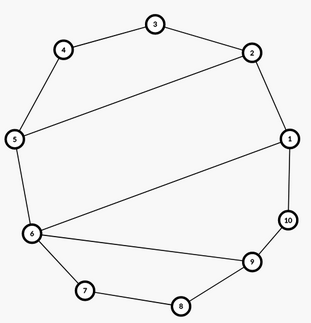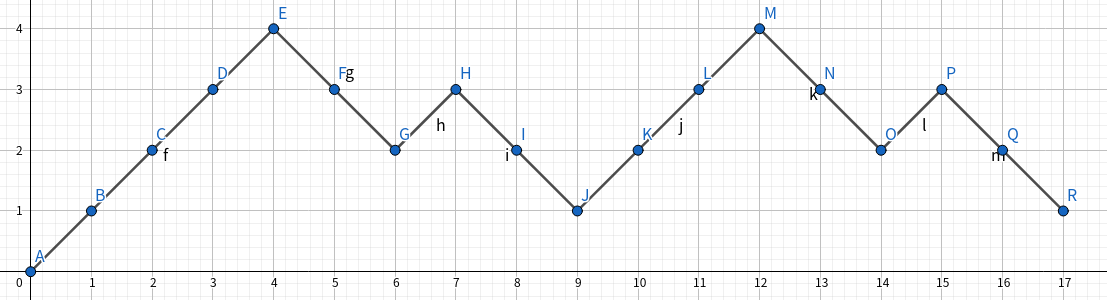# [笔记] 卡特兰数

### 基础知识

#### 卡特兰数的式子

\begin{aligned} &h(n)=\sum_{i=0}^{n-1}h(i)\cdot h(n-i-1)\\ &h(n)=\frac{h(n-1)\cdot(4n-2)}{n+1}\\ &h(n)=\frac{\binom{2n}{n}}{n+1}=\frac{\binom{2n+1}{n}}{2n+1} \end{aligned}

#### 卡特兰数的例子

• 合法出栈入栈操作序列。
• 合法括号序列。
• 凸多边形划分为三角形的方案数。

### 拓展

#### 广义卡特兰数

$$m$$ 边凸多边形划分为 $$n$$$$k$$ 边形的方案 ( $$m=nk-2(n-1)$$ )

$h(n)=\sum_{S}\prod_{x\in S} h(x)\ [\ |S|=k-1,\sum_{x\in S}x=n-1]$

$H(n)=xH^{k-1}(n)+1$$$1$$ 号点出发，依次遍历所有顶点，每抵达一个顶点，记为 $$+1$$，若当前顶点有指向编号更小的顶点的弦，则把划分出的一块全部割掉，那么此时边数的变化是减少了 $$k-1$$ 条边，然后增加了当前弦这一条边，故记为 $$-(k-2)$$#### Raney 引理

posted @ 2022-05-27 14:55  IrisT  阅读(34)  评论(0编辑  收藏  举报